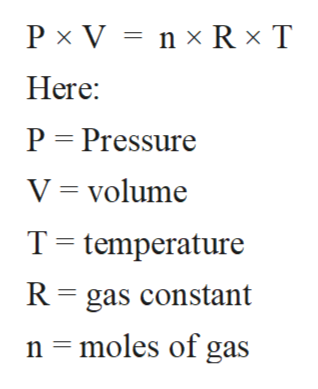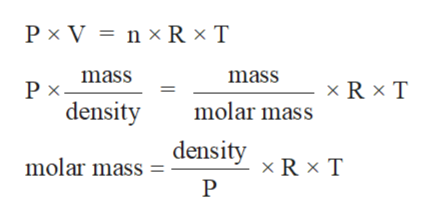# Determine the molecular weight (molar mass) of gases at STP which have densities of: 0.090 g/L

Question
20 views

Determine the molecular weight (molar mass) of gases at STP which have densities of: 0.090 g/L

check_circle

Step 1

The gaseous state is the state of matter with least intermolecular force of attraction between molecules and highest kinetic energy. The standard properties of gases like Pressure, volume, and temperature can be calculated with the help of ideal gas equation.

The ideal gas equation can be written as:help_outlineImage TranscriptioncloseP x V Here n x R xT P Pressure V volume T temperature R gas constant n moles of gas fullscreen
Step 2

Density and molar mass can also calculate with the help of ideal gas equation:help_outlineImage TranscriptioncloseP x V n x R x T mass mass R x T Рх. density molar mass density molar mass xR x T P fullscreen
Step 3

Given:

Density = 0.090 g/L

R = 0.0821 L.atm/K.mol

T = 273...

### Want to see the full answer?

See Solution

#### Want to see this answer and more?

Solutions are written by subject experts who are available 24/7. Questions are typically answered within 1 hour.*

See Solution
*Response times may vary by subject and question.
Tagged in

### Ideal gases